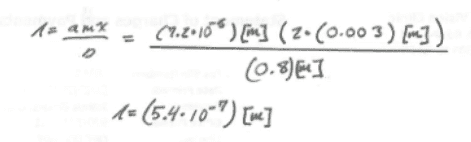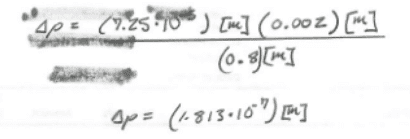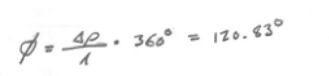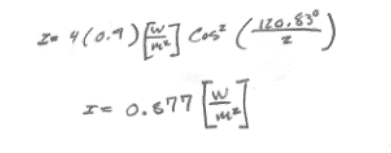# Intensity and Interference Patterns (double slit)

• garthenar

#### garthenar

Homework Statement
Two slits spaced 0.0720 mm apart are 0.800 m from a screen. Coherent light of wavelength λ passes through the two slits. In their interference pattern on the screen, the distance from the center of the central maximum to the first minimum is 3.00 mm. The intensity at the peak of the central maximum is 0.0900 W/m2.

a) What is the intensity at point on the screen that is 2.00 mm from the center of the central maximum?

b) What is the intensity at point on the screen that is 1.50 mm from the center of the central maximum?
Relevant Equations
λ=(ax)/D
a = distance between the two slits
x = the distance between maximums
m = which maximum your looking at (from the center)
D = the distance between the "source" (slits) and the screen

path difference
Δp = asin(θ)
= atan(θ)
= (ay/D)
y = vertical distance

phase difference
Φ = Δp (2π / λ)
= Δp k

I = 4(I_0)cos^2(Φ/2)
I'm still on part a.
I think that i may have the wrong equation for intensity.
I'm not sure I'm using the right numbers for the "first minimum".

I started with getting the wavelength

λ=(ax)/D
since the first minimum occurs at m = 0.5 I multiplied the distance to the first minimum by 2 to get the distance to the first "fringe". (point of maximum constructive interference)I then got the path difference

Δp = (ay/D)
I used the distance to the point I want the intensity at for yThen the phase difference

Φ = Δp (2π / λ)And finally my intensity

I = 4(I_0)cos^2(Φ/2)Which was wrong.
Can you help me figure out where I went wrong. I've tried several variation on this.

Thank you

#### Attachments

Your work looks good up to here:
And finally my intensity

I = 4(I_0)cos^2(Φ/2)
View attachment 261531
Be sure you understand the meaning of I_0 in the formula. Is it the intensity of the central max of the double-slit pattern, or is it the intensity that one slit alone would produce at y = 0 on the screen?

Your work looks good up to here:
Be sure you understand the meaning of I_0 in the formula. Is it the intensity of the central max of the double-slit pattern, or is it the intensity that one slit alone would produce at y = 0 on the screen?
Wait, I though the central max was at y =0. Where is the central max?

Wait, I though the central max was at y =0. Where is the central max?
Yes, the central max is at y = 0. The question is, what does the symbol I_0 represent in the formula?

Yes, the central max is at y = 0. The question is, what does the symbol I_0 represent in the formula?
I'm not entirely sure. My professor said that I should use the equation (I_0)cos^2(Φ/2) with I_0 equaling the intensity at the origin of the two slit model. So I'm assuming that the 4(I_0) has something to do with the the intensity one slit would produce. We haven't done single slits so I'll have to go check that out.

I'm not entirely sure. My professor said that I should use the equation (I_0)cos^2(Φ/2) with I_0 equaling the intensity at the origin of the two slit model. So I'm assuming that the 4(I_0) has something to do with the the intensity one slit would produce. We haven't done single slits so I'll have to go check that out.
Yes. In the 4(I_0) formula, I_0 is the intensity that one slit alone would produce at the location of the central max. The total amplitude at the central maximum due to both slits is twice the amplitude due to one slit alone. Intensity is proportional to the square of the amplitude. So, the intensity of the central max due to both slits together is 4 times the intensity that one slit alone would produce.

If you leave out the factor of 4 in the formula, then I_0 would be the intensity of the central max due to both slits together.